Courses

# Capacitors in Series and Parallel Physics Notes | EduRev

## Physics : Capacitors in Series and Parallel Physics Notes | EduRev

The document Capacitors in Series and Parallel Physics Notes | EduRev is a part of the Physics Course Basic Physics for IIT JAM.
All you need of Physics at this link: Physics

Capacitance in Series

(a) shows a series connection of three capacitors with a voltage applied. As for any capacitor, the capacitance of the combination is related to charge and voltage by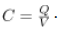Note that opposite charges of magnitude Q flow to either side of the originally uncharged combination of capacitors when the voltage V is applied. Conservation of charge requires that equal-magnitude charges be created on the plates of the individual capacitors, since charge is only being separated in these originally neutral devices. The end result is that the combination resembles a single capacitor with an effective plate separation greater than that of the individual capacitors alone. (See (b).) Larger plate separation means smaller capacitance. It is a general feature of series connections of capacitors that the total capacitance is less than any of the individual capacitances.

(a) Capacitors connected in series. The magnitude of the charge on each plate is  Q. (b) An equivalent capacitor has a larger plate separation d. Series connections produce a total capacitance that is less than that of any of the individual capacitors.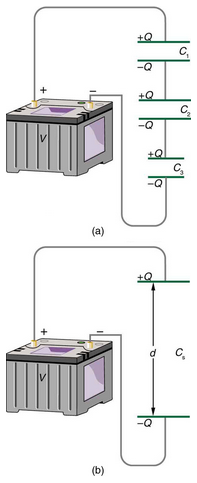We can find an expression for the total capacitance by considering the voltage across the individual capacitors shown in. Solving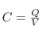for V gives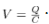The voltages across the individual capacitors are thus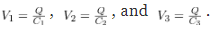The total voltage is the sum of the individual voltages:

V = V1 + V2 + V3.

Now, calling the total capacitance Cs for series capacitance, consider that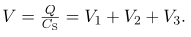Entering the expressions for V1 , V2 and V3, we get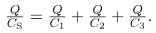Canceling the Qs, we obtain the equation for the total capacitance in series Cs to be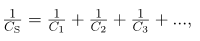where “…” indicates that the expression is valid for any number of capacitors connected in series. An expression of this form always results in a total capacitance Cs that is less than any of the individual capacitances C1,C2, …, as the next example illustrates.

Total Capacitance in Series, Cs

Total capacitance in series: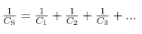What Is the Series Capacitance?

Find the total capacitance for three capacitors connected in series, given their individual capacitances are 1.000, 5.000, and 8.000 F.

Strategy

With the given information, the total capacitance can be found using the equation for capacitance in series.

Solution

Entering the given capacitances into the expression for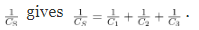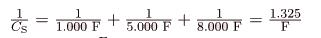Inverting to find Cs yields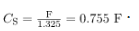Discussion

The total series capacitance Cs is less than the smallest individual capacitance, as promised. In series connections of capacitors, the sum is less than the parts. In fact, it is less than any individual. Note that it is sometimes possible, and more convenient, to solve an equation like the above by finding the least common denominator, which in this case (showing only whole-number calculations) is 40. Thus,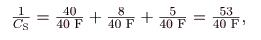so that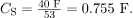Capacitors in Parallel

(a) shows a parallel connection of three capacitors with a voltage applied. Here the total capacitance is easier to find than in the series case. To find the equivalent total capacitance Cp, we first note that the voltage across each capacitor is V, the same as that of the source, since they are connected directly to it through a conductor. (Conductors are equipotentials, and so the voltage across the capacitors is the same as that across the voltage source.) Thus the capacitors have the same charges on them as they would have if connected individually to the voltage source. The total charge Q is the sum of the individual charges: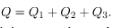(a) Capacitors in parallel. Each is connected directly to the voltage source just as if it were all alone, and so the total capacitance in parallel is just the sum of the individual capacitances. (b) The equivalent capacitor has a larger plate area and can therefore hold more charge than the individual capacitors.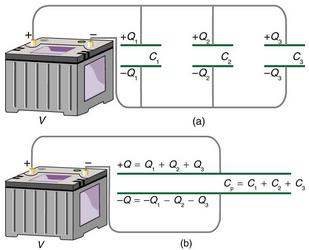Using the relationship Q= CV, we see that the total charge is Q= CpV, and the individual charges are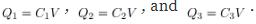and Q3 = C3V. Entering these into the previous equation gives

CpV = C1V + C2V +C3V.

Canceling V from the equation, we obtain the equation for the total capacitance in parallel Cp

Cp = C1 + C2+C3+....

Total capacitance in parallel is simply the sum of the individual capacitances. (Again the “…” indicates the expression is valid for any number of capacitors connected in parallel.) So, for example, if the capacitors in the example above were connected in parallel, their capacitance would be

Cp = 1.000 F + 5.000 F + 8.000 F +  14.000 F.

The equivalent capacitor for a parallel connection has an effectively larger plate area and, thus, a larger capacitance, as illustrated in (b).

Total Capacitance in Parallel, Cp

Total capacitance in parallel Cp = C1 + C2+C3+....

More complicated connections of capacitors can sometimes be combinations of series and parallel. (See) To find the total capacitance of such combinations, we identify series and parallel parts, compute their capacitances, and then find the total.

(a) This circuit contains both series and parallel connections of capacitors. for the calculation of the overall capacitance of the circuit. (b) C1 and C2 are in series; their equivalent capacitance Cs is less than either of them. (c) Note that Cs is in parallel with C3. The total capacitance is, thus, the sum of Cs and C3.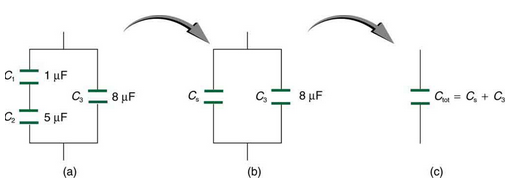A Mixture of Series and Parallel Capacitance
Find the total capacitance of the combination of capacitors shown in [link]. Assume the capacitances in [link] are known to three decimal places (C1=1.000F ’ C2 = 5.000F’ and C3= 8.000F), and round your answer to three decimal places.

Strategy

To find the total capacitance, we first identify which capacitors are in series and which are in parallel. Capacitors C1 and C2 are in series. Their combination, labeled Cs in the figure, is in parallel with C3.

Solution

Since C1 and C2 are in series, their total capacitance is given by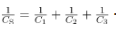Entering their values into the equation gives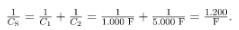Inverting gives

Cs = 0.833 F.

This equivalent series capacitance is in parallel with the third capacitor; thus, the total is the sum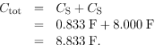Discussion

This technique of analyzing the combinations of capacitors piece by piece until a total is obtained can be applied to larger combinations of capacitors.

Section Summary

• Total capacitance in series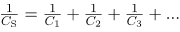• Total capacitance in parallel Cp = C1 + C2+C3+....
• If a circuit contains a combination of capacitors in series and parallel, identify series and parallel parts, compute their capacitances, and then find the total.

Conceptual Questions

If you wish to store a large amount of energy in a capacitor bank, would you connect capacitors in series or parallel? Explain.

Problems & Exercises

Find the total capacitance of the combination of capacitors.

A combination of series and parallel connections of capacitors.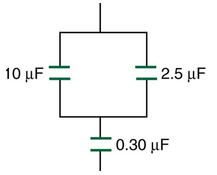0.293 F

Suppose you want a capacitor bank with a total capacitance of 0.750 F and you possess numerous 1.50 mF capacitors. What is the smallest number you could hook together to achieve your goal, and how would you connect them?

What total capacitances can you make by connecting a 5.00 F and an 8.00 F capacitor together?

3.08 F in series combination, 13.0 F in parallel combination

Find the total capacitance of the combination of capacitors.

A combination of series and parallel connections of capacitors.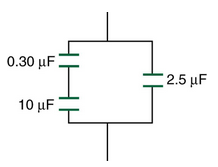2.79 F

Find the total capacitance of the combination of capacitors.

A combination of series and parallel connections of capacitors.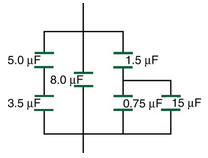Unreasonable Results

(a) An 8.00 F capacitor is connected in parallel to another capacitor, producing a total capacitance of 5.00 F. What is the capacitance of the second capacitor? (b) What is unreasonable about this result? (c) Which assumptions are unreasonable or inconsistent?

(a) -3.00 F
(b) You cannot have a negative value of capacitance.
(c) The assumption that the capacitors were hooked up in parallel, rather than in series, was incorrect. A parallel connection always produces a greater capacitance, while here a smaller capacitance was assumed. This could happen only if the capacitors are connected in series.

Offer running on EduRev: Apply code STAYHOME200 to get INR 200 off on our premium plan EduRev Infinity!

## Basic Physics for IIT JAM

109 videos|157 docs|94 tests

,

,

,

,

,

,

,

,

,

,

,

,

,

,

,

,

,

,

,

,

,

;